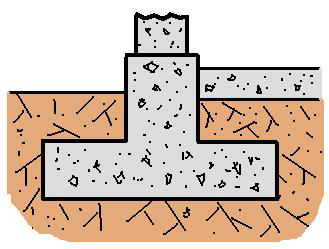* Log In to use the Calculate function * Become a Member!

###### Design of Shallow Foundations:• Calculate the required area of a spread footing foundation.

Square Footing Uplift Resistance
• Calculate the uplift resistance of a square footing foundation.
• Validate the trial design of a spread footing foundation.
Required Pier Diameter
• Calculate the diameter required for a pier foundation.
Round Footing Uplift Resistance
• Calculate the uplift resistance of a round or circular foundation.
Validate Pier Design
• Validate the trial design for a pier foundation.
Required Lat Ld Pier Depth
• Calculate the required depth of pier for a lateral load.
• Perform iterative design analysis to find the required depth of steel for 1-way shear of a spread footing.
• Perform iterative design analysis to find the required depth of steel for 2-way shear of a spread footing.
• Calculate the required depth of steel for a wall load on a spread footing.